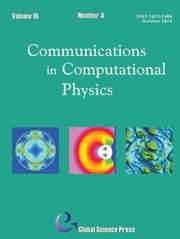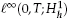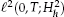Home
Hostname: page-component-544b6db54f-8tjh8 Total loading time: 0.178 Render date: 2021-10-16T16:35:49.852Z Has data issue: true Feature Flags: { "shouldUseShareProductTool": true, "shouldUseHypothesis": true, "isUnsiloEnabled": true, "metricsAbstractViews": false, "figures": true, "newCiteModal": false, "newCitedByModal": true, "newEcommerce": true, "newUsageEvents": true }Communications in Computational Physics

# An Efficient, Energy Stable Scheme for the Cahn-Hilliard-Brinkman System

Published online by Cambridge University Press:  03 June 2015

*
Corresponding author.Email:swise@math.utk.edu

## Abstract

We present an unconditionally energy stable and uniquely solvable finite difference scheme for the Cahn-Hilliard-Brinkman (CHB) system, which is comprised of a Cahn-Hilliard-type diffusion equation and a generalized Brinkman equation mod-eling fluid flow. The CHB system is a generalization of the Cahn-Hilliard-Stokes model and describes two phase very viscous flows in porous media. The scheme is based on a convex splitting of the discrete CH energy and is semi-implicit. The equations at the implicit time level are nonlinear, but we prove that they represent the gradient of a strictly convex functional and are therefore uniquely solvable, regardless of time step size. Owing to energy stability, we show that the scheme is stable in the time and space discreteandnorms. We also present an efficient, practical nonlinear multigrid method . comprised of a standard FAS method for the Cahn-Hilliard part, and a method based on the Vanka smoothing strategy for the Brinkman part . for solving these equations. In particular, we provide evidence that the solver has nearly optimal complexity in typical situations. The solver is applied to simulate spinodal decomposition of a viscous fluid in a porous medium, as well as to the more general problems of buoyancy- and boundary-driven flows.

Type
Research Article
Information
Communications in Computational Physics , April 2013 , pp. 929 - 957

## Access options

Get access to the full version of this content by using one of the access options below. (Log in options will check for institutional or personal access. Content may require purchase if you do not have access.)

## References

Bertozzi, A.L., Esedoglu, S., and Gillette, A.Inpainting of binary images using the Cahn- Hilliard equation. IEEE Trans. Image Proc., 16:285291,2007.CrossRefGoogle ScholarPubMed
Brinkman, H.C.A calculation of the viscous force exerted by a flowing fluid on a dense swarm of particles. Appl. Sci. Res., AI:2734,1949.CrossRefGoogle Scholar
Cahn, J.W.On spinodal decomposition. Acta Metall., 9:795801,1961.CrossRefGoogle Scholar
Cahn, J.W., Elliott, C.M., and Novick-Cohen, A.The Cahn-Hilliard equation with a concentration dependent mobility: Motion by minus the Laplacian of the mean curvature. Euro. J. Appl. Math., 7:287301,1996.CrossRefGoogle Scholar
Cahn, J.W. and Hilliard, J.E.Free energy of a nonuniform system. I. Interfacial free energy. J. Chem. Phys., 28:258267,1958.Google Scholar
Elliott, C.M. and Stuart, A.M.The global dynamics of discrete semilinear parabolic equations. SIAM J. Numer. Anal, 30:16221663,1993.CrossRefGoogle Scholar
Eyre, D.Unconditionally gradient stable time marching the Cahn-Hilliard equation. In Bullard, J. W., Kalia, R., Stoneham, M., and Chen, L.Q., editors, Computational and Mathematical Models of Microstructural Evolution, volume 53, pages 16861712, Warrendale, PA, USA, 1998. Materials Research Society.Google Scholar
Feng, X.Fully discrete finite element approximations of the Navier-Stokes-Cahn-Hilliard diffuse interface model for two-phase fluid flows. SIAM J. Numer. Anal., 44:10491072, 2006.CrossRefGoogle Scholar
Feng, X. and Wise, S.M.Analysis of a Darcy-Cahn-Hilliard diffuse interface model for the Hele-Shaw flow and its fully discrete finite element approximation. SIAM J. Numer. Anal., 50(3), 1320V1343,2012.CrossRefGoogle Scholar
Hu, Z., Wise, S.M., Wang, C., and Lowengrub, J.S.Stable and efficient finite-difference nonlinear-multigrid schemes for the phase-field crystal equation. J. Comput. Phys., 228:53235339,2009.CrossRefGoogle Scholar
Kay, D. and Welford, R.A multigrid finite element solver for the Cahn-Hilliard equation. J. Comput. Phys., 212:288304,2006.CrossRefGoogle Scholar
Kay, D. and Welford, R.Efficient numerical solution of Cahn-Hilliard-Navier Stokes fluids in 2D. SIAM J. Sci. Comput., 29:22412257,2007.CrossRefGoogle Scholar
Kim, J.S., Kang, K., and Lowengrub, J.S.Conservative multigrid methods for Cahn-Hilliard fluids. J. Comput. Phys., 193:511543,2003.CrossRefGoogle Scholar
Lee, H.G., Lowengrub, J.S, and Goodman, J.Modeling pinchoff and reconnection in a Hele-Shaw cell. I. The models and their calibration. Phys. Fluids, 14:492513,2002.Google Scholar
Lee, H.G., Lowengrub, J.S, and Goodman, J.Modeling pinchoff and reconnection in a Hele-Shaw cell. II. Analysis and simulation in the nonlinear regime. Phys. Fluids, 14:514545, 2002.Google Scholar
Liu, C. and Shen, J.A phase field model for the mixture of two incompressible fluids and its approximation by a Fourier-spectral method. Physica D, 179:211228,2003.CrossRefGoogle Scholar
Lowengrub, J.S. and Truskinovsky, L.Cahn-Hilliard fluids and topological transitions. Proc. R. Soc. Lond. A, 454:26172654,1998.CrossRefGoogle Scholar
Ngamsaad, W., Yojina, J., and Triampo, WTheoretical studies of phase-separation kinetics in a Brinkman porous medium. J. Phys. A: Math. Theor., 43:202001,2010.CrossRefGoogle Scholar
Oosterlee, C.W. and Gaspar, F.J.Multigrid relaxation methods for systems of saddle point type. Appl. Numer. Math., 58:19331950,2008.CrossRefGoogle Scholar
Pham, K., Frieboes, H.B., Cristini, V., and Lowengrub, J.Predictions of tumour morphological stability and evaluation against experimental observations. J. R. Soc. Interface, 8:1629,2011.CrossRefGoogle ScholarPubMed
Shen, J., Wang, C., Wang, X., and Wise, S.Second-order convex splitting schemes for gradient flows with Ehrlich-Schwoebel-type energy: Application to thin film epitaxy. SIAM J. Numer. Anal., 50:105125,2012.CrossRefGoogle Scholar
Shen, J. and Yang, X.Energy stable schemes for Cahn-Hilliard phase-field model of two- phase incompressible flows. Chinese Ann. Math. Series B, 31:743758,2010.CrossRefGoogle Scholar
Shen, J. and Yang, X.Numerical approximations of Allen-Cahn and Cahn-Hilliard equations. Discrete Cont. Dyn. Sys. A, 28:16691691,2010.CrossRefGoogle Scholar
Shen, J. and Yang, X.A phase-field model and its numerical approximation for two-phase incompressible flows with different densities and viscosities. SIAM J. Sci. Comput., 32:11591179, 2010.CrossRefGoogle Scholar
Trottenberg, U., Oosterlee, C.W., and Schuller, A.Multigrid. Academic Press, New York, 2005.Google Scholar
Vanka, S.P.Block-implicit multigrid solution of Navier-Stokes equations in primitive variables. J. Comput. Phys., 65:138158,1986.CrossRefGoogle Scholar
Vollmayr-Lee, B.P. and Rutenberg, A.D.Fast and accurate coarsening simulation with an unconditionally stable time step. Phys. Rev. E, 68:066703, 2003.CrossRefGoogle ScholarPubMed
Wang, C., Wang, X., and Wise, S.Unconditionally stable schemes for equations of thin film epitaxy. Discrete Cont. Dyn. Sys. A, 28:405423,2010.Google Scholar
Wang, C. and Wise, S.Global smooth solution of modified phase field crystal equation. Methods Appl. Anal., 17:191212,2010.Google Scholar
Wang, C. and Wise, S.An energy stable and convergent finite-difference scheme for the modified phase field crystal equation. SIAM J. Numer. Anal., 49:945969,2011.CrossRefGoogle Scholar
Wise, S.M.Unconditionally stable finite difference, nonlinear multigrid simulation of the Cahn-Hilliard-Hele-Shaw system of equations. J. Sci. Comput., 44:3868,2010.CrossRefGoogle Scholar
Wise, S.M., Lowengrub, J.S., and Cristini, V.An adaptive algorithm for simulating solid tumor growth using mixture models. Math. Comput. Model., 53:120,2011.CrossRefGoogle ScholarPubMed
Wise, S.M., Lowengrub, J.S., Frieboes, H.B., and Cristini, V.Three-dimensional multispecies nonlinear tumor growth -1 model and numerical method. J. Theor. Biol., 253:524543,2008.CrossRefGoogle Scholar
Wise, S.M., Wang, C., and Lowengrub, J.S.An energy stable and convergent finite-difference scheme for the phase field crystal equation. SIAM J. Numer. Anal., 47:22692288,2009.CrossRefGoogle Scholar
47
Cited by

# Send article to Kindle

Note you can select to send to either the @free.kindle.com or @kindle.com variations. ‘@free.kindle.com’ emails are free but can only be sent to your device when it is connected to wi-fi. ‘@kindle.com’ emails can be delivered even when you are not connected to wi-fi, but note that service fees apply.

Find out more about the Kindle Personal Document Service.

An Efficient, Energy Stable Scheme for the Cahn-Hilliard-Brinkman System
Available formats
×

# Send article to Dropbox

To send this article to your Dropbox account, please select one or more formats and confirm that you agree to abide by our usage policies. If this is the first time you use this feature, you will be asked to authorise Cambridge Core to connect with your <service> account. Find out more about sending content to Dropbox.

An Efficient, Energy Stable Scheme for the Cahn-Hilliard-Brinkman System
Available formats
×

# Send article to Google Drive

To send this article to your Google Drive account, please select one or more formats and confirm that you agree to abide by our usage policies. If this is the first time you use this feature, you will be asked to authorise Cambridge Core to connect with your <service> account. Find out more about sending content to Google Drive.

An Efficient, Energy Stable Scheme for the Cahn-Hilliard-Brinkman System
Available formats
×
×